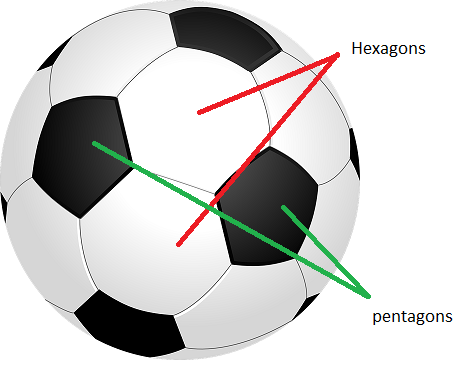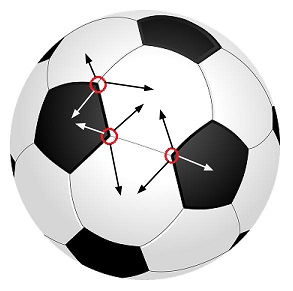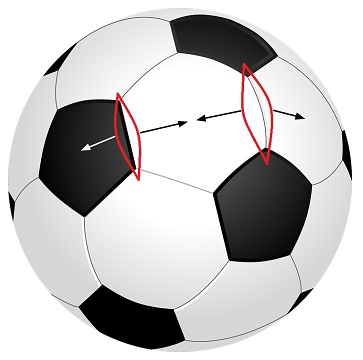Skip to content
Related Articles
Number of Pentagons and Hexagons on a Football
• Difficulty Level : Hard
• Last Updated : 25 Feb, 2018

Given a standard football, regular hexagons and pentagons are drawn on it as shown in the picture. Find out the number of hexagons and pentagons.## Recommended: Please try your approach on {IDE} first, before moving on to the solution.

We can apply Euler Characteristics to find out number of Hexagons and Pentagons on a standard Football.
According to Euler Characteristics: For every surface S there exists an integersuch that whenever a graph G with V vertices and E edges is embedded in S so that there are F faces(regions divided by the graph), we have:For a sphere(shape of football)= 2.
Hence, the equation becomes V – E + F = 2.

Now, let number of pentagons P and number of hexagons H.

Number of vertices will be:
6 vertices for each of the hexagons, i.e. 6*H.
5 vertices for each of the pentagons, i.e. 5*P.
But we have counted each vertex thrice, once for each adjacent polygon, follow the pictureHence, number of vertices, V = (6*H + 5*P)/3.

Number of edges will be:
6 edges for each of the hexagons, i.e. 6*H.
5 edges for each of the pentagons, i.e. 5*P.
But we have counted each edge twice, once for each adjacent polygon, follow the pictureHence, number of edges, E = (6*H + 5*P)/2.

Number of faces will be:
There are H hexagons and P pentagons, each forming a face. Hence, total number of faces, F = (H + P).

So, we can write:after solving this equation we will get P = 12. So, there are 12 Pentagons.
Now number of Hexagons:
We can see that each pentagon is surrounded by 5 Hexagons. So there should 5*P hexagons, but we have counted each hexagon thrice for each of its 3 adjacent pentagons. Hence, number of hexagons = 5*P/3 = 5*12/3 = 20.

Hence, there are 20 Hexagons and 12 Pentagons in a standard football.

Attention reader! Don’t stop learning now. Get hold of all the important DSA concepts with the DSA Self Paced Course at a student-friendly price and become industry ready.  To complete your preparation from learning a language to DS Algo and many more,  please refer Complete Interview Preparation Course.

In case you wish to attend live classes with industry experts, please refer DSA Live Classes

My Personal Notes arrow_drop_up